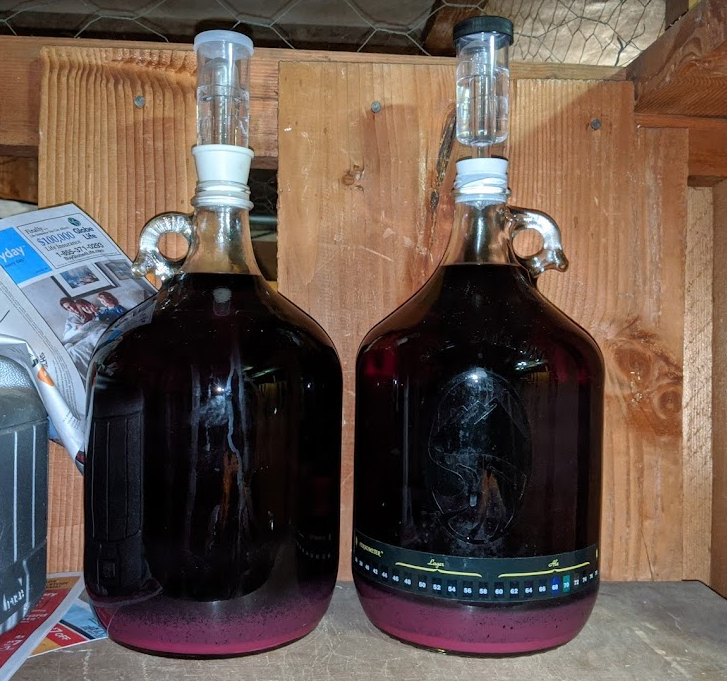# Chaptalization Calculator

### 2021

When making fruit wine, you have to add sugar to get a wine-like alcohol content. Blackberry juice, for example, normally has a specific gravity of only 1.03-1.04. My process when making wine is to measure the volume and SG of the juice and then determine how much sugar to add to get a SG of around 1.08 in the final five gallons of must. The problem is that all the calculators I could find only tell you how much sugar to add to your final volume — adding sugar at this point will obviously result in more volume after it dissolves. Home brewing doesn't require a lot of precision, but I was still annoyed that I could not find a simple calculator to tell me how much sugar to add to, say, three gallons of must such that after adding water to reach, say, five gallons the SG would be a specific value, so I made one.Blackberry Wine

This works by determining how much sugar there is to begin with and how much sugar is needed at the end based on the specified specific gravity values. Amount of water to add is not really needed since you can add until the desired volume is reahced, but I included the estimate for completeness. Degrees Plato is a measure of how many grams of sucrose are in 100 grams of solution. The same definition is used for degrees Brix and the two scales can be considered equal for most purposes. Using the estimated °P and known starting SG, sugar quantity can be estimated by multiplying by the starting mass of the must by its °P. The Starting mass is just the starting volume multiplied by the stating specific gravity.

To determine degrees Plato from the specific gravity, I use a second order approximation published by R. Lincoln in Computer compatible parametric equations for basic brewing computation Master Brewers Association of the Americas Technical Quarterly 24 (1987) 129-132:

$\mathrm{°P\left(SG\right)}=\left(\mathrm{\left(463},-,205SG\right)\right)\left(\mathrm{\left(SG},-,1\right)\right)$

These calculations assume that all sugar in solution is sucrose which works out reasonably for brewing as other sugars have similar SG to mass relationships. The estimated final alcohol content equation assumes that all dissolved solids are fermentable.